# Breast Cancer Detection with Machine Learning | The Future of Healthcare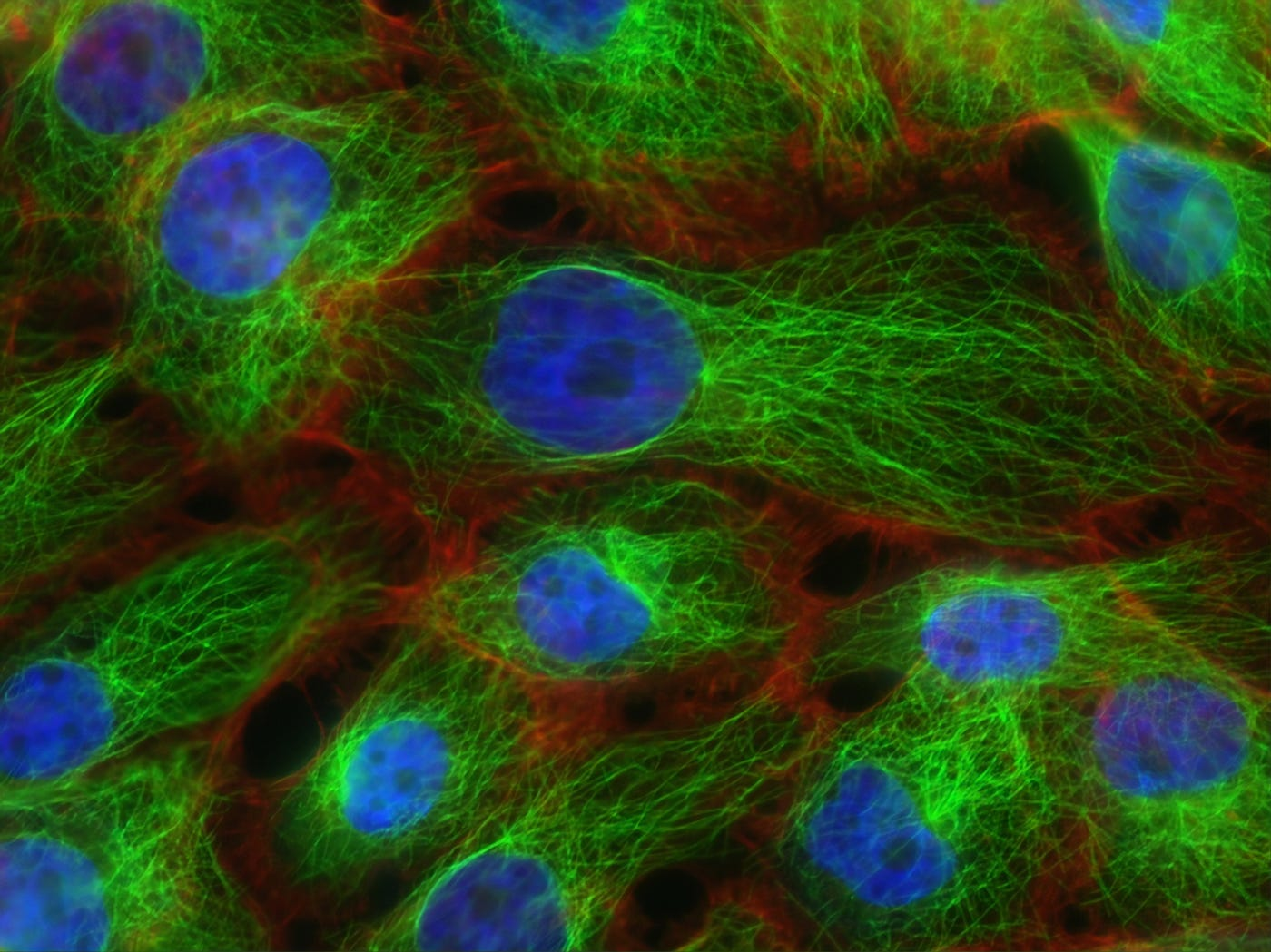Photo by National Cancer Institute on Unsplash

Health is among the many things that we take for granted in our daily lives. When we become ill or start getting diseases, the pain, the process of diagnosis, the treatment, is all that’s expected. What we often don’t think about is the backbone that allows all of that to happen, the healthcare system. The doctors, the infrastructure, the hospital itself, along with all of the machines that power the diagnosis, and the treatment. This is the way it’s been done for many decades and it’s had great success.

# Here’s How Breast Cancer Is Currently Diagnosed and Treated

Current Screening Guidelines:

Typically for breast cancer, doctors and healthcare practitioners use mammograms (a type of x-ray), ultrasounds, MRI’s and/or biopsies to help diagnose patients.

A mammogram is the most important, and most frequently used, as it’s an x-ray of the breast. It can detect cancerous cells up to two years earlier than a tumour can be felt by the patient or the doctor.

For early cancer detection, the American Cancer Society recommends that:

• “Women age 45 to 54 should get mammograms every year” and that…
• “Women 55 and older should switch to mammograms every 2 years or can continue yearly screening.”

If the patient is diagnosed with breast cancer, doctors then explore treatments to get rid of cancer, which depends on the “type of breast cancer, its stage and grade, size, and whether the cancer cells are sensitive to hormones” says the Mayo Clinic.

Doctors would then proceed with either removing the breast cancer (lumpectomy), removing the entire breast (mastectomy), removing a limited number of lymph nodes (sentinel node biopsy), removing several lymph nodes (axillary lymph node dissection) or removing both breasts. As well depending on the situation and a variety of factors, other treatments also exist, such as radiation therapy, chemotherapy, hormone therapy, immunotherapy, and more. (Mayo Clinic).

Infinite advances in medicine have led up to the point at which we are today, with endless tools and talent to help diagnose and treat one of the most common types of cancer among women, one that affected over 2 million worldwide in 2018 alone (WCRF).

# Wait… I Had A Shower Thought Though…I’m working on that skincare routine…

I distinctly remember it being at 8 in the morning during the holiday break. I was taking a shower and my mind wandered to medicine and how grateful I was for the whole healthcare system, and how it’s taken care of so many of my loved ones, and me.

Somehow, my mind magically wandered and then made the connection with my fascination with AI to it being used to either help treat or diagnose illnesses. After I finished washing up, I grabbed my phone from the counter and started taping away, researching projects and datasets on Kaggle, trying to see what I can do as part of my next project in surrounding Artificial Intelligence. And then… BAM. I found it.

The “Breast Cancer Wisconsin (Diagnostic) Data Set.”

The project’s prompt? “Predict whether the cancer is benign or malignant”

Then I promptly threw the project idea into my to-do list on Notion and started looking into how I could go about this. Hours later, I got started. Here’s the process, and the walkthrough.

# The Project.

I had a number of steps ahead of me, but I went one by one, determined to complete it. Here’s the process, and the walkthrough.

## Step 0: Data Preparation.

The dataset that we’ll be using today is publically available here and here.

It’s been put together by the Department of Machine Learning at UCI (University of California, Irvine). Inside, it includes a list of features from digitized images of a fine needle aspirate (FNA) of a breast mass, which was gathered by Dr. William H. Wolberg, W. Nick Street, and Olvi L. Mangasarian from the University of Wisconsin.

Dr. William H. Wolberg created the dataset using fluid samples taken from patients with solid breast masses and a piece of software called Xcyt, which was able to analyze cytological features based on his digital scans. ‘The program then uses a curve-fitting algorithm, computing 10 features from each cell in the sample, calculating the mean value, extreme value, and standard error of each feature for the image, returning a 30 real-valuated vector.’ (V. Goel).

Here’s some of the dataset’s pulled directly from the site:

Attribute Information:

1) ID number
2) Diagnosis (M = malignant, B = benign)
3–32) Feat

Ten real-valued features are computed for each cell nucleus:

a) radius (mean of distances from center to points on the perimeter)
b) texture (standard deviation of gray-scale values)
c) perimeter
d) area
e) smoothness (local variation in radius lengths)
f) compactness (perimeter² / area — 1.0)
g) concavity (severity of concave portions of the contour)
h) concave points (number of concave portions of the contour)
i) symmetry
j) fractal dimension (“coastline approximation” — 1)

Huge shoutout to all the computer scientists, doctors and researchers at both universities who put this together! Another shoutout goes to the Youtube channel, ‘Computer Science’ for providing valuable help on this project when I got stuck!

## Step 1: Data Exploration

The first step as part of any project is opening the IDE, or environment that you’ll be using. I opened a Jupyter notebook on Google Colab and started working away. The first step was to explore the data to see what I was working with and here’s the breakdown of what I did:

First, I imported the key libraries and packages needed for the project.

`import numpy as np import pandas as pd import matplotlib.pyplot as plt import seaborn as sns`

Next up, I downloaded the dataset and fed it into the program. I also printed the first 8 rows of data to understand what I was working with.

`*#Load the dataset into the program*from google.colab import filesuploaded = files.upload()df = pd.read_csv('data.csv')df.head(8)`

As you can, there are a variety of features that were included, and in the second column, you can see the confirmed diagnosis of each patient.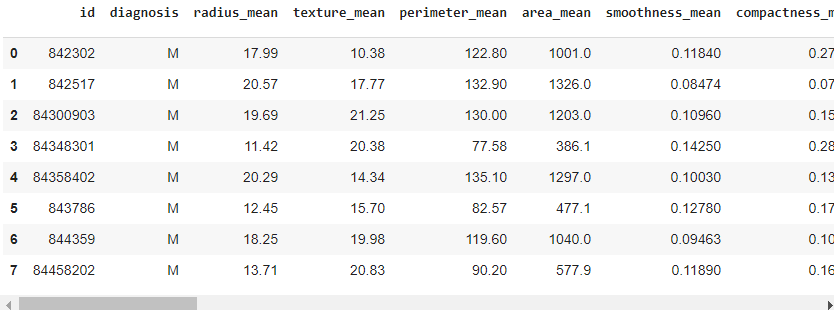Sample of the first 8 patients in the dataset.

Next, I decided to look at the data, and figure out the number of rows and columns in my dataset. I realized that there were indeed 569 rows of data, meaning that 569 patients were represented in this dataset and that there were 33 columns of data surrounding each patient, 31 if you exclude the patient id and the confirmed diagnosis.

`#Count the number of rows and columns in the data setdf.shape`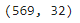(Number of Rows, Number of Columns)

I wanted to clean up any useless parts in my data, and try to find any columns that were empty or didn’t contain anything (NaN, NAN, na) values.

`#Count the number of empty (NaN, NAN, na) values in each columndf.isna().sum()`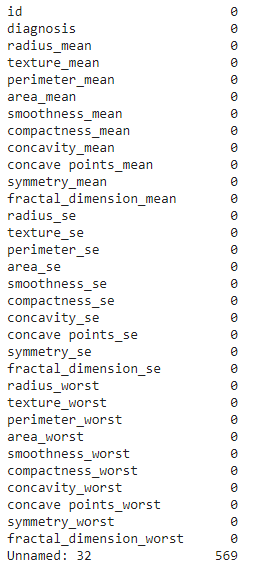Counts of the number of empty values in each column

As a result, I removed the last column, since it had no meaning.

`#Drop the column with empty values (Na, NAN, NaN)df = df.dropna(axis=1)`

Then I got the new count of the number of rows and columns to confirm that the last one was excluded.

`#Get the new count of the number of rows and columnsdf.shape`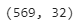Updated to include only 569 rows and 32 columns.

Next, I wanted to know how many of the patients had malignant cancerous cells (M) and benign (B) non-cancerous cells. As well, I wanted to visualize it, and create a count plot.

`#Get a count of the number of the Malignant (M) and Benign (B) cellsdf['diagnosis'].value_counts()#Visualize the countsns.countplot(df['diagnosis'], label='count')`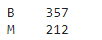# of Malignant Diagnoses: 212 and # of Benign Diagnoses: 357

To make sure that all the data types are correct, I ran some code to see if there were any non-numerical data, which showed the column ‘diagnosis’ was categorical data, an object in Python.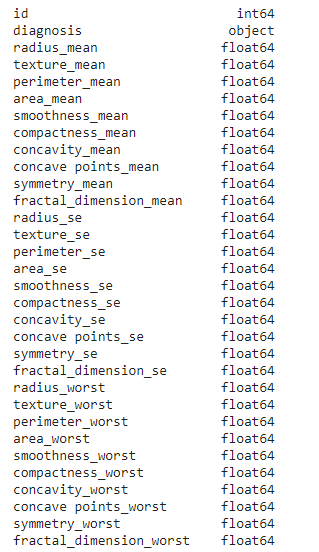List of columns and data types

# Step 2 — Categorical Data

The data set included categorical data which are variables that are label values instead of numerical values. Examples of categorical data use cases would include referencing country, gender, age group, etc. As a result, I wanted to turn the ‘diagnosis’ values into 1 and 0, from M and B, respectively, and then print the results. In other words, using the Label Encoder, I converted Benign to 0 and Malignant to 1.

`#Encoding categorical data values (from sklearn.preprocessing import LabelEncoderlabelencoder_Y = LabelEncoder()df.iloc[:,1]= labelencoder_Y.fit_transform(df.iloc[:,1].values)print(labelencoder_Y.fit_transform(df.iloc[:,1].values))`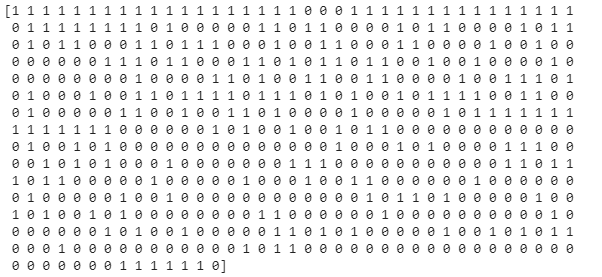Here are the encoded values of the column, ‘diagnosis.’

Next, I decided to visualize the data, to understand it better, and create scatter plots where variables in the same data row are matched with one another. Remember that Benign = 0 and Malignant = 1.

`#Create a pair plot, remember that 0 means benign and 1 means malignantsns.pairplot(df.iloc[:,1:5], hue = 'diagnosis')`

Now that I could visualize and see the correlations, I also wanted to let the computer display the numerical correlations.

`#Get the correlatioins of the columnsdf.iloc[:,1:12].corr()`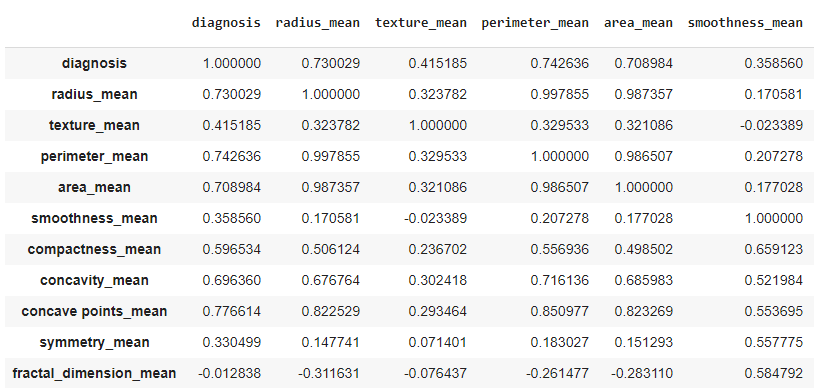A small sample of the numerical representations of correlations

I also wanted to visualize the correlation by creating a heat map. I also wanted to change the values so that it would display how likely variables were correlated in percentages instead of a 0–1 scale.

`#Visualize the correlationplt.figure(figsize=(10,10))sns.heatmap(df.iloc[:,1:12].corr(), annot=True , fmt='.0%')`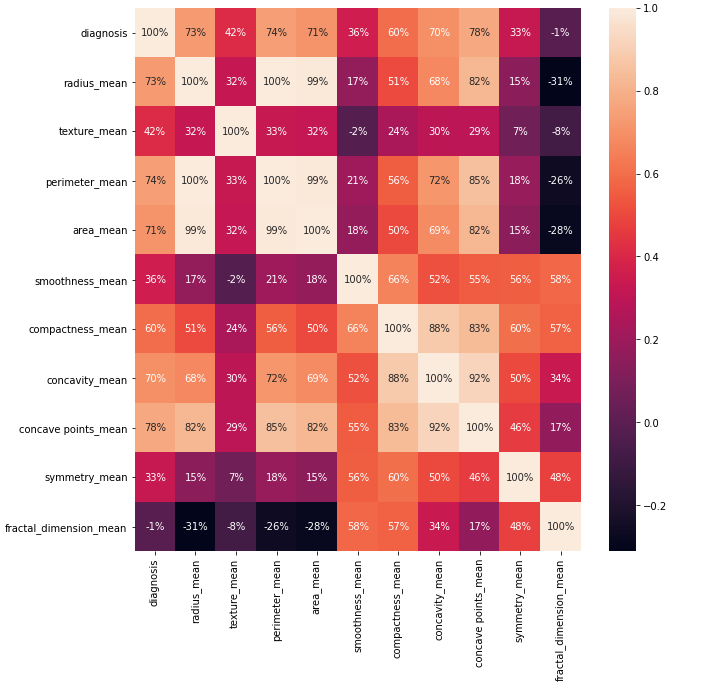Quick heat map of the correlations

# Step 3 — Feature Scaling and Splitting Data

After exploring, and cleaning up the data, I set up the data by splitting the data set into the feature data set (independent data set, with variable X) and the target data set (dependant data set, with variable Y)

`X = df.iloc[:, 2:31].values Y = df.iloc[:, 1].values`

Then I split it again to have 75% allocated for training and 25% used for testing when I’ve completed the program.

`#Splitting Datafrom sklearn.model_selection import train_test_splitX_train, X_test, Y_train, Y_test = train_test_split(X, Y, test_size = 0.25, random_state = 0)`

Now the goal is to scale up the data with feature scaling to ensure that all features are the same level of magnitude. This means that the feature or independent data will be within a specific range from 0–1 or 0–100.

`#Feature Scalingfrom sklearn.preprocessing import StandardScalersc = StandardScaler()X_train = sc.fit_transform(X_train)X_test = sc.transform(X_test)`

# Step 4 — Model Selection

At this point, we’ve gotten to the most exciting part of the project, where we’ll be applying a bunch of Machine Learning algorithms to see which one provides the best results during prediction.

In the Machine Learning world, data scientists use many types of Machine Learning algorithms, but from a bigger picture, they are usually divided into two groups, supervised learning and unsupervised learning.

Supervised Learning
This is a technique in which we teach or train the machine using data that is well labelled. Furthermore, this labelled dataset helps to train the model to understand patterns in the data. This can be further divided into two types of problems: Regression (where the output is a continuous value, i.e. salary, house prices, weight, age), and Classification (where the output is a category, i.e. similar to operating like binary, outputting either 0 or 1, or outputting “correct” or “incorrect”, etc.)

Unsupervised Learning
This is a technique where we have an algorithm use data that is unlabeled and unclassified. In this case, the algorithm needs to do its best to draw its own connections, conclusions and predictions.

In our case, this problem of determining whether a cancer cell is malignant or benign will call for a Supervised Learning Classification Algorithm.

Now, lastly, I decided to test a number of machine learning models to see which one works best for this classification program, by creating and calling a function. These models include Logistic Regression, Decision Tree Classifier, and Random Forest Classifier.

`#Create a function for the modelsdef models(X_train, Y_train):#Logistic Regression  from sklearn.linear_model import LogisticRegression  log = LogisticRegression(random_state=0)  log.fit(X_train, Y_train)#Decision Tree  from sklearn.tree import DecisionTreeClassifier  tree = DecisionTreeClassifier(criterion = 'entropy', random_state=0)  tree.fit(X_train, Y_train)#Random Forest Classifier  from sklearn.ensemble import RandomForestClassifier  forest = RandomForestClassifier(n_estimators = 10, criterion = 'entropy', random_state=0)  forest.fit(X_train, Y_train)#Print the model's accuracy on the training data  print(' Logistic Regression Training Accuracy', log.score(X_train, Y_train))  print(' Decision Tree Classifier Training Accuracy', tree.score(X_train, Y_train))  print(' Random Forest Classifier Training Accuracy', forest.score(X_train, Y_train))return log, tree, forest# Getting all of the modelsmodel = models(X_train, Y_train)`

Here are the results, and accuracy rates. It seems as though the accuracy rates are 99.0%, 100.0%, and 99.5%, for the Logistic Regression, Decision Tree, and Random Forest models, respectively.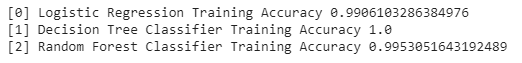Note: Multiply the value by 100 to get the percentage accuracy.

Next, I’m also going to try and test model accuracy on the test data with the Confusion Matrix. This would show us how many patients each model misdiagnosed.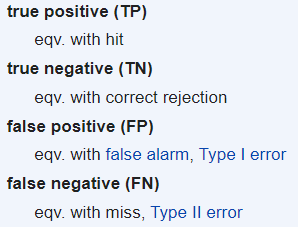Confusion Matrix Table and Explanations, Source: Wikipedia
`#Test Model Accuracy on Test Data on Confusion Matrixfrom sklearn.metrics import confusion_matrixfor i in range( len(model) ):   print('Model', i)  cm = confusion_matrix(Y_test, model[i].predict(X_test))TN = cm  TP = cm  FN = cm  FP = cmprint(cm)  print('Testing Accuracy = ', (TP + TN) / (TP + TN + FN + FP))  print()`

Lastly, to confirm our metrics, I ran another method of testing the accuracy rate of the model.

`# Alternative Method to Get Metrics of The Modelsfrom sklearn.metrics import classification_reportfrom sklearn.metrics import accuracy_scorefor i in range(len(model)):   print('Model', i)  print()  print(classification_report(Y_test, model[i].predict(X_test)))  print(accuracy_score(Y_test, model[i].predict(X_test)))`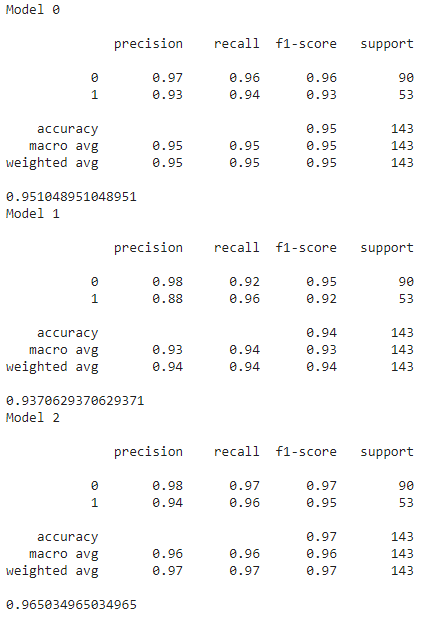Metrics on the accuracy rates and the metrics on the test data (25% of the original dataset size)

Based on the numbers from above, we can see that the model that performs the best is indeed the Random Forest Classifier with an accuracy rate of 96.5%! Not too shabby in the context of Machine Learning, but if this were to be used on real patients, the accuracy rate would need to be much higher, since this would mean that for every 100 patients this model runs predictions on, it’ll get it wrong on 3.5 patients. A bit more tunning of these models would be necessary if used in real medical settings. Hopefully, with further advancements, we’ll get to a point where accuracy rates basically hit 100%.

As well, since I was curious, I wanted to see which patients that the Random Forest Classifier model messed up on, or didn’t do too well at. As you can see there are a few mistakes that the model made, particularly in the first row.

`#Print the prediction of Random Forest Classifier Modelpred = model.predict(X_test)print(pred) #predictionsprint()print(Y_test) #real diagnosis according to the original dataset`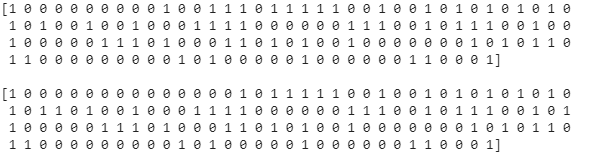The final comparison between the model’s predictions (top), vs. the real diagnosis according to the original dataset (bottom).

This was an extremely fun project and I hope you enjoyed this quick walkthrough of what I did! In the years to come, I hope that the computer science communities continue to work alongside nurses, doctors and researchers to help push our boundaries and help decrease the time of diagnosing patients, saving many more lives in the process.

## Resources

Feel free to drop any comments down below if you had any questions or wanted to provide any feedback or suggestions.

As well, here are some links down below on where you can find me if that’s your cup of tea:

--

--## Kevin Liu

16-year-old TKS Innovator, and AI Enthusiast, working on developing a legendary skillset to solve the world’s most important problems